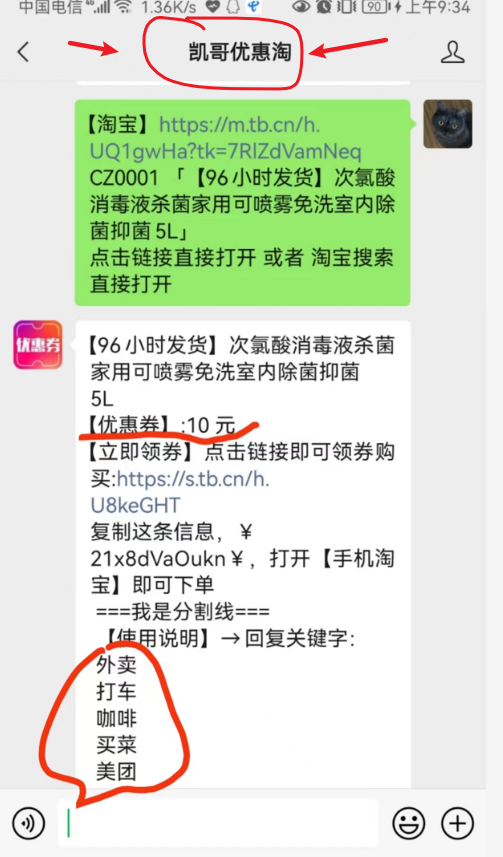### CAS的缺点和问题解决

• 并发
• 时间：2020-03-22 12:58
• 1878人已阅读ABA：

```   public long sum() {
Cell[] as = cells; Cell a;        long sum = base;        if (as != null) {            for (int i = 0; i < as.length; ++i) {                if ((a = as[i]) != null)
sum += a.value;
}
}        return sum;
}```TopTop